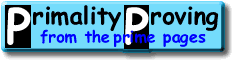Finding primes & proving primality Chapter 2: The quick tests for small numbers and probable primesHome > Primality Proving > Chapter Two (contents)

### Contents of this Chapter:

This is one of four chapters on finding primes and proving primality.  The first is a short introduction and table of contents.  The second (these pages) chapter discusses finding small primes and the basic probable primality tests.  The third chapter cover the classical primality tests that have been used to prove primality for 99.99% of the numbers on the largest known prime list.  The last chapter introduces the general purpose tests that do not require factorization.

 [ next page | previous page ] Copyright © 2023 PrimePages (Originally written by Chris Caldwell)